##### Physics I For Dummies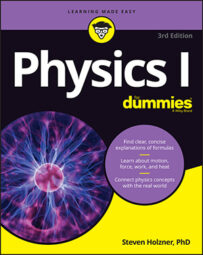When a satellite travels in a geosynchronous orbit around the Earth, it needs to travel at a certain orbiting radius and period to maintain this orbit. Because the radius and period are related, you can use physics to calculate one if you know the other.

The period of a satellite is the time it takes it to make one full orbit around an object. The period of the Earth as it travels around the sun is one year. If you know the satellite’s speed and the radius at which it orbits, you can figure out its period.

You can calculate the speed of a satellite around an object using the equation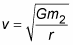The satellite travels around the entire circumference of the circle — which is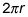if r is the radius of the orbit — in the period, T. This means the orbital speed must be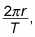giving you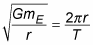If you solve this for the period of the satellite, you get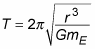You, the intuitive physicist, may be wondering: What if you want to examine a satellite that simply stays stationary over the same place on the Earth at all times? In other words, a satellite whose period is the same as the Earth’s 24-hour period? Can you do it?

Such satellites do exist. They’re very popular for communications because they’re always orbiting in the same spot relative to the Earth; they don’t disappear over the horizon and then reappear later. They also allow for the satellite-based global positioning system, or GPS, to work.

In cases of stationary satellites, the period, T, is 24 hours, or about 86,400 seconds. Can you find the distance a stationary satellite needs be from the center of the Earth (that is, the radius) to stay stationary? Using the equation for periods, you see thatPlugging in the numbers, you get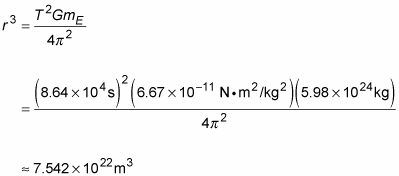If you take the cube root of this, you get a radius of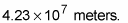This is the distance the satellite needs to be from the center of the Earth. Subtracting the Earth’s radius of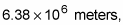you get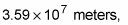which converts to about 22,300 miles. This is the distance from the surface of the Earth geosynchronous satellites need to orbit. At this distance, they orbit the Earth at the same rate the Earth is turning, which means that they stay put over the same piece of real estate.

In practice, it’s very hard to get the speed just right, which is why geosynchronous satellites have either gas boosters that can be used for fine-tuning or magnetic coils that allow them to move by pushing against the Earth’s magnetic field.